• +91 9971497814
• info@interviewmaterial.com

# RD Chapter 6- Graphs of Trigonometric Functions Ex-6.1 Interview Questions Answers

### Related Subjects

Question 1 :
Sketch the graphs of the following functions:
(i) f (x) = 2 sin x, 0 ≤ x ≤ π
(ii) g (x) = 3 sin (x – π/4), 0 ≤ x ≤ 5π/4
(iii) h (x) = 2 sin 3x, 0 ≤ x ≤ 2π/3
(iv) ϕ (x) = 2 sin (2x – π/3), 0 ≤ x ≤ 7π/3
(v) Ψ (x) = 4 sin 3 (x – π/4), 0 ≤ x ≤ 2π
(vi) θ (x) = sin (x/2 – π/4), 0 ≤ x ≤ 4π
(vii) u (x) = sin2 x, 0 ≤ x ≤ 2π υ (x) = |sin x|, 0 ≤ x ≤ 2π
(viii) f (x) = 2 sin πx, 0 ≤ x ≤ 2

(i) f (x) = 2 sin x, 0 ≤ x ≤ π
We know that g (x) = sin x is a periodic function with period π.
So, f (x) = 2 sin x is a periodic function with period π. So, we will draw the graph of f (x) = 2 sin x in the interval [0, π]. The values of f (x) = 2 sin x at various points in [0, π] are listed in the following table:
 x 0(A) π/6 (B) π/3 (C) π/2 (D) 2π/3 (E) 5π/6 (F) Π (G) f (x) = 2 sin x 0 1 √3 = 1.73 2 √3 = 1.73 1 0

The required curve is: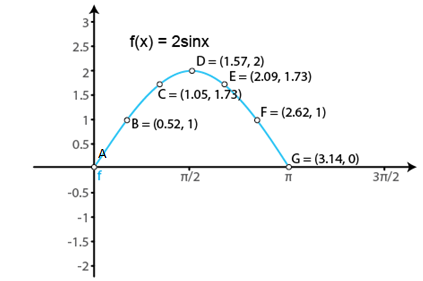(ii) g (x) = 3 sin (x – π/4), 0 ≤ x ≤ 5π/4

We know that if f (x) isa periodic function with period T, then f (ax + b) is periodic with periodT/|a|.

So, g (x) = 3 sin (x –π/4) is a periodic function with period π. So, we will draw the graph of g (x)= 3 sin (x – π/4) in the interval [0, 5π/4]. The values of g (x) = 3 sin(x – π/4) at various points in [0, 5π/4] are listed in the following table:

 x 0(A) π/4 (B) π/2 (C) 3π/4 (D) π (E) 5π/4 (F) g (x) = 3 sin (x – π/4) -3/√2 = -2.1 0 3/√2 = 2.12 3 3/√2 = 2.12 0

The required curve is: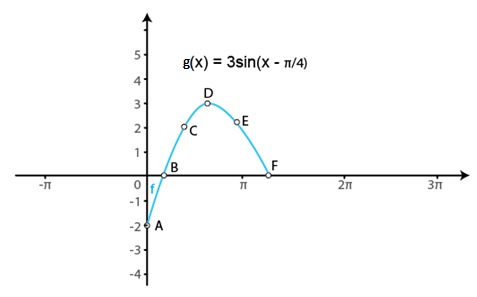(iii) h (x) = 2 sin 3x, 0 ≤ x ≤ 2π/3

We know that g (x) = sinx is a periodic function with period 2π.

So, h (x) = 2 sin 3x isa periodic function with period 2π/3. So, we will draw the graph of h (x) = 2sin 3x in the interval [0, 2π/3]. The values of h (x) = 2 sin 3x at variouspoints in [0, 2π/3] are listed in the following table:

 x 0 (A) π/6 (B) π/3 (C) π/2 (D) 2π/3 (E) h (x) = 2 sin 3x 0 2 0 -2 0

The required curve is: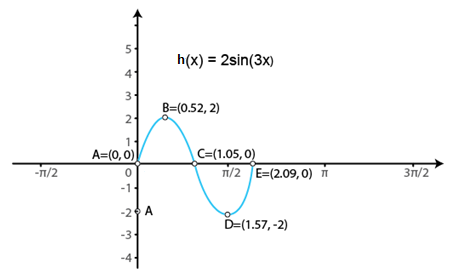(iv) ϕ (x) = 2 sin (2x – π/3), 0 ≤ x ≤ 7π/3

We know that if f(x) isa periodic function with period T, then f (ax + b) is periodic with periodT/|a|.

So, ϕ (x) = 2 sin (2x – π/3) is a periodicfunction with period π. So, we will draw the graph of ϕ (x) = 2 sin (2x –π/3), in the interval [0, 7π/5]. The values of ϕ (x) = 2 sin (2x –π/3), at various points in [0, 7π/5] are listed in the following table:

 x 0 (A) π/6 (B) 2π/3 (C) 7π/6 (D) 7π/5 (E) ϕ (x) = 2 sin (2x – π/3) -√3 = -1.73 0 0 0 1.98

The required curve is: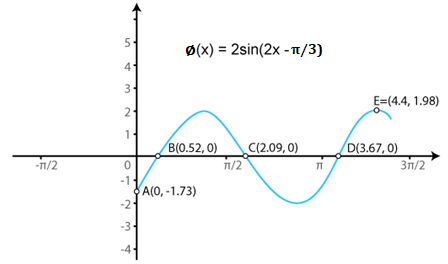(v) Ψ (x) = 4 sin 3 (x – π/4), 0 ≤ x ≤ 2π

We know that if f(x) isa periodic function with period T, then f (ax + b) is periodic with periodT/|a|.

So, Ψ (x) = 4 sin 3(x – π/4) is a periodic function with period 2π. So, we will draw thegraph of Ψ (x) = 4 sin 3 (x – π/4) in the interval [0, 2π]. The values ofΨ (x) = 4 sin 3 (x – π/4) at various points in [0, 2π] are listed in thefollowing table:

 x 0 (A) π/4 (B) π/2 (C) π (D) 5π/4 (E) 2π (F) Ψ (x) = 4 sin 3 (x – π/4) -2√2 = -2.82 0 2√2 = 2.82 0 1.98 -2√2 = -2.82

The required curve is: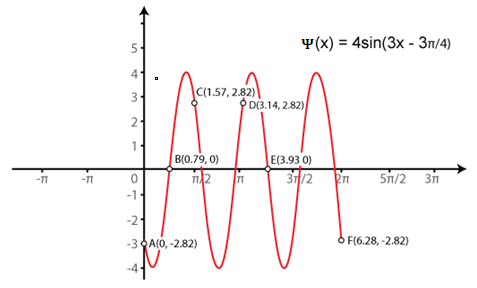(vi) θ (x) = sin (x/2 – π/4), 0 ≤ x ≤ 4π

We know that if f(x) isa periodic function with period T, then f (ax + b) is periodic with periodT/|a|.

So, θ (x) = sin (x/2 –π/4) is a periodic function with period 4π. So, we will draw the graphof θ (x) = sin (x/2 – π/4) in the interval [0, 4π]. The valuesof θ (x) = sin (x/2 – π/4) at various points in [0, 4π] are listed in thefollowing table:

 x 0 (A) π/2 (B) π (C) 2π (D) 5π/2 (E) 3π (F) 4π (G) θ (x) = sin (x/2 – π/4) -1/√2 = -0.7 0 1/√2 = 0.7 1/√2 = 0.7 0 -1/√2 = -0.7 -1/√2 = -0.7

The required curve is: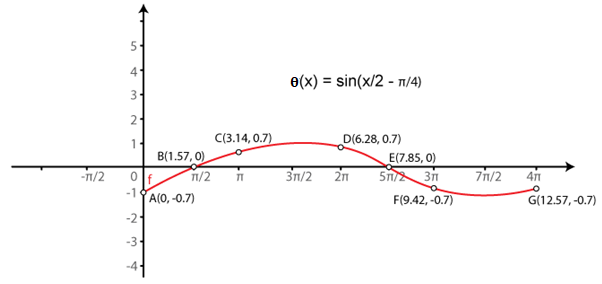(vii) u (x) = sin2 x,0 ≤ x ≤ 2π υ (x) = |sin x|, 0 ≤ x ≤ 2π

We know that g (x) = sinx is a periodic function with period π.

So, u (x) = sin2 x is a periodic function withperiod 2π. So, we will draw the graph of u (x) = sin2 xin the interval [0, 2π]. The values of u (x) = sin2 xat various points in [0, 2π] are listed in the following table:

 x 0 (A) π/2 (B) Π (C) 3π/2 (D) 2π (E) u (x) = sin2 x 0 1 0 1 0

The required curve is: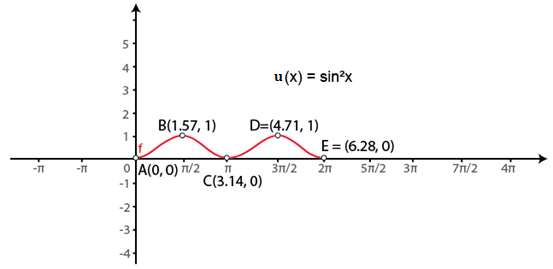(viii) f (x) = 2 sin πx, 0 ≤ x ≤ 2

We know that g (x) = sinx is a periodic function with period 2π.

So, f (x) = 2 sin πx isa periodic function with period 2. So, we will draw the graph of f (x) = 2 sinπx in the interval [0, 2]. The values of f (x) = 2 sin πx at various points in[0, 2] are listed in the following table:

 x 0 (A) 1/2 (B) 1 (C) 3/2 (D) 2 (E) f (x) = 2 sin πx 0 2 0 -2 0

The required curve is: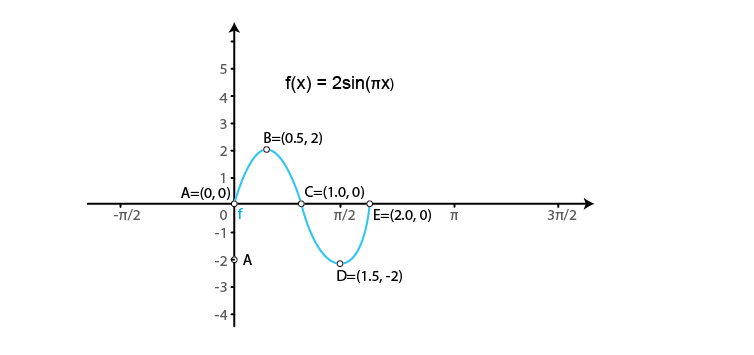Question 2 :
Sketch the graphs of the following pairs of functions on the same axes:
(i) f (x) = sin x, g (x) = sin (x + π/4)
(ii) f (x) = sin x, g (x) = sin 2x
(iii) f (x) = sin 2x, g (x) = 2 sin x
(iv) f (x) = sin x/2, g (x) = sin x

(i) f (x) = sin x, g (x) = sin (x + π/4)
We know that the functions f (x) = sin x and g (x) = sin (x + π/4) are periodic functions with periods 2π and 7π/4.
The values of these functions are tabulated below:
Values of f (x) = sin x in [0, 2π]
 x 0 π/2 π 3π/2 2π f (x) = sin x 0 1 0 -1 0

Values of g (x) = sin (x + π/4) in [0, 7π/4]

 x 0 π/4 3π/4 5π/4 7π/4 g (x) = sin (x + π/4) 1/√2 = 0.7 1 0 -1 0

The required curve is: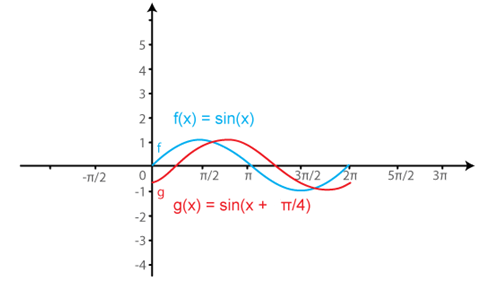(ii) f (x) = sin x, g (x) = sin 2x

We know that the functions f(x) = sin x and g (x) = sin 2x are periodic functions with periods 2π and π.

The values of these functions are tabulated below:

Values of f (x) = sin x in [0, 2π]

 x 0 π/2 π 3π/2 2π f (x) = sin x 0 1 0 -1 0

Values of g (x) = sin (2x) in [0, π]

 x 0 π/4 π/2 3π/4 π 5π/4 3π/2 7π/4 2π g (x) = sin (2x) 0 1 0 -1 0 1 0 -1 0

The required curve is: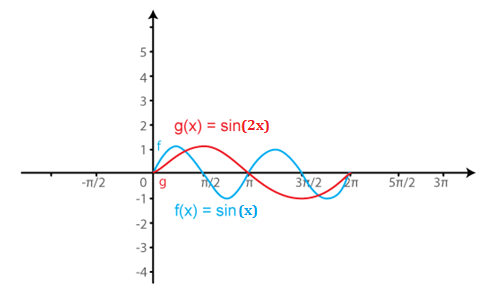(iii) f (x) = sin 2x, g (x) = 2 sin x

We know that the functions f(x) = sin 2x and g (x) = 2 sin x are periodic functions with periods π and π.

The values of these functions are tabulated below:

Values of f (x) = sin (2x) in [0, π]

 x 0 π/4 π/2 3π/4 π 5π/4 3π/2 7π/4 2π f (x) = sin (2x) 0 1 0 -1 0 1 0 -1 0

Values of g (x) = 2 sin x in [0, π]

 x 0 π/2 π 3π/2 2π g (x) = 2 sin x 0 1 0 -1 0

The required curve is: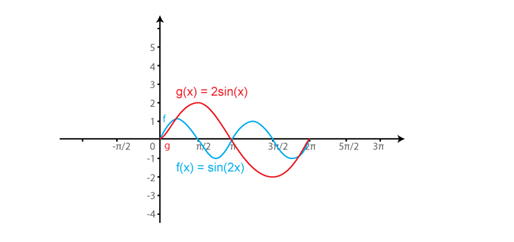(iv) f (x) = sin x/2, g (x) = sin x

We know that the functions f(x) = sin x/2 and g (x) = sin x are periodic functions with periods π and 2π.

The values of these functions are tabulated below:

Values of f (x) = sin x/2 in [0, π]

 x 0 π 2π 3π 4π f (x) = sin x/2 0 1 0 -1 0

Values of g (x) = sin (x) in [0, 2π]

 x 0 π/2 π 3π/2 2π 5π/2 3π 7π/2 4π g (x) = sin (x) 0 1 0 -1 0 1 0 -1 0

The required curve is: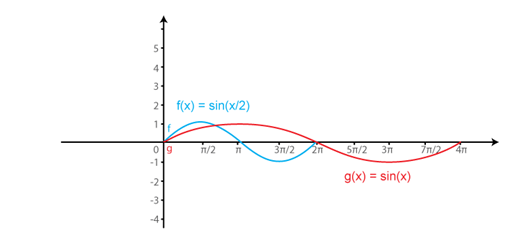Todays Deals### RD Chapter 6- Graphs of Trigonometric Functions Ex-6.1 Contributorskrishan

Name:
Email:

# Latest News# 9000 interview questions in different categories